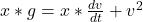## In a rocket-propulsion problem the mass is variable. Another such problem is a raindrop falling through a cloud of small water droplets. Som

Question

In a rocket-propulsion problem the mass is variable. Another such problem is a raindrop falling through a cloud of small water droplets. Some of these small droplets adhere to the raindrop, thereby increasing its mass as it falls. The force on the raindrop is
Fext=dp/dt=m dv/dt+v dm/dt
Suppose the mass of the raindrop depends on the distance x that it has fallen. Then m = kx, where k is a constant, and dm/dt=kv
dm/dt=kv This gives, since Fext=mg
Fext​=mg,
mg=m dv/dt+v(kv)
Or, dividing by k,
xg=x dv/dt+v2
This is a differential equation that has a solution of the form v = at, where a is the acceleration and is constant. Take the initial velocity of the raindrop to be zero.
(a) Using the proposed solution for v find the acceleration a.
(b) Find the distance the raindrop has fallen in t = 3.00 s.
(c) Given that k = 2.00 g/m, find the mass of the raindrop at t = 3.00 s.

in progress 0
2 months 2021-08-02T09:25:49+00:00 1 Answers 8 views 0

a) a = g / 3

b) x (3.0) = 14.7 m

c) m (3.0) = 29.4 g

Explanation:

Given:-

– The following differential equation for (x) the distance a rain drop has fallen has the form:– Where,                v = Speed of the raindrop

– Proposed solution to given ODE:

v = a*t

Where,                  a = acceleration of raindrop

Find:-

(a) Using the proposed solution for v find the acceleration a.

(b) Find the distance the raindrop has fallen in t = 3.00 s.

(c) Given that k = 2.00 g/m, find the mass of the raindrop at t = 3.00 s.

Solution:-

– We know that acceleration (a) is the first derivative of velocity (v):

a = dv / dt   … Eq 1

– Similarly, we know that velocity (v) is the first derivative of displacement (x):

v = dx / dt  , v = a*t … proposed solution (Eq 2)

v .dt = dx = a*t . dt

– integrate both sides:

∫a*t . dt = ∫dt

x = 0.5*a*t^2  … Eq 3

Substitute Eq1 , 2 , 3 into the given ODE:

0.5*a*t^2*g = 0.5*a^2 t^2 + a^2 t^2

= 1.5 a^2 t^2

a = g / 3

– Using the acceleration of raindrop (a) and t = 3.00 second and plug into Eq 3:

x (t) = 0.5*a*t^2

x (t = 3.0) = 0.5*9.81*3^2 / 3

x (3.0) = 14.7 m

– Using the relation of mass given, and k = 2.00 g/m, determine the mass of raindrop at time t = 3.0 s:

m (t) = k*x (t)

m (3.0) = 2.00*x(3.0)

m (3.0) = 2.00*14.7

m (3.0) = 29.4 g